# 1.0 Physical Quantities and Measurement

10 Jul 2016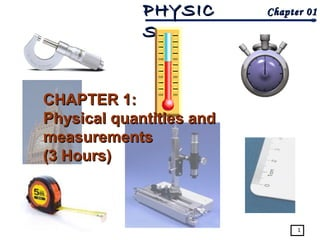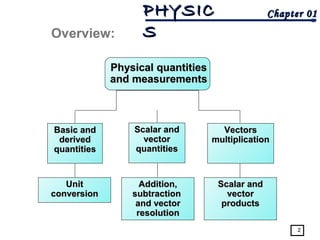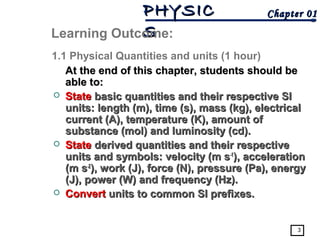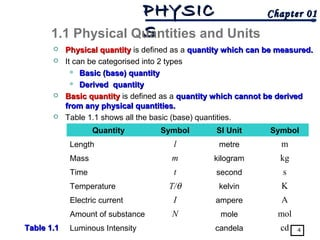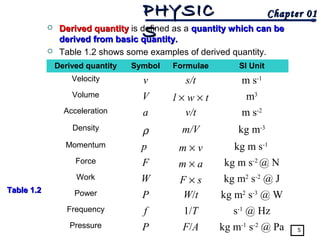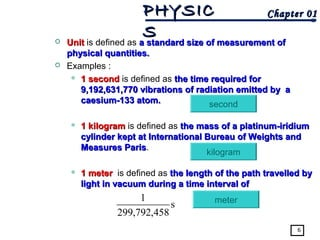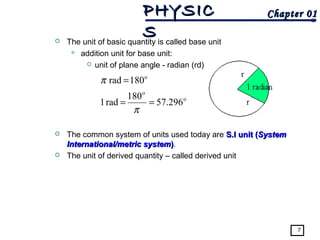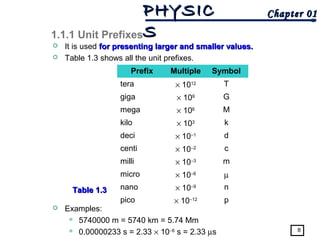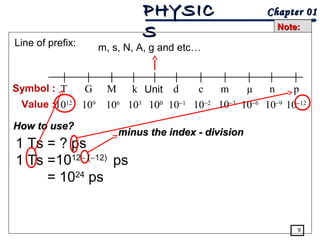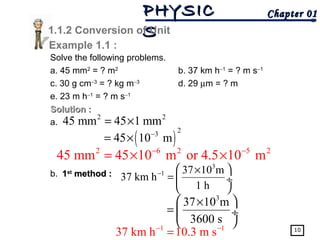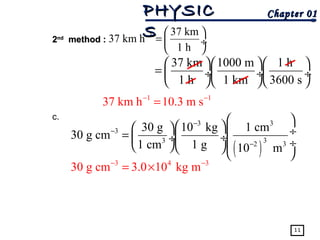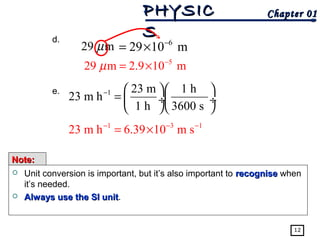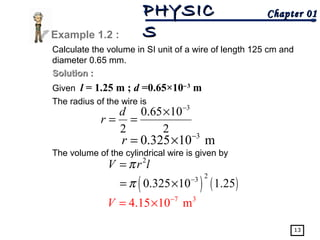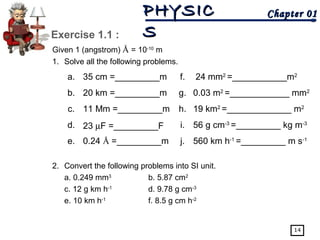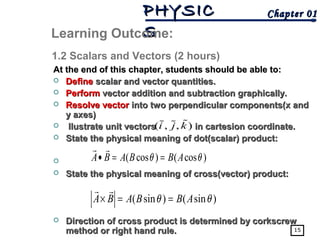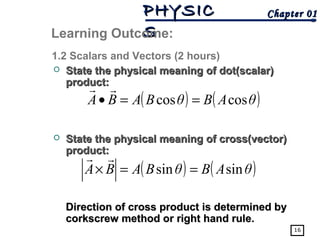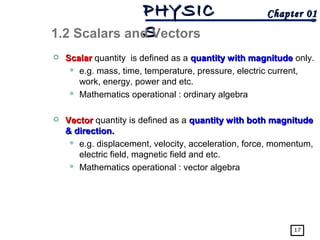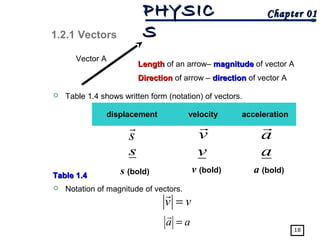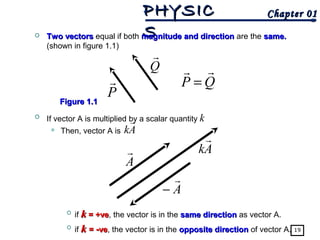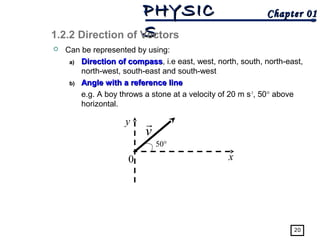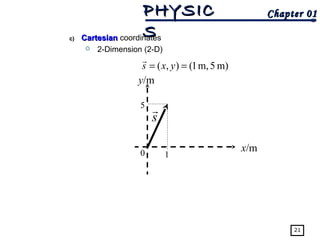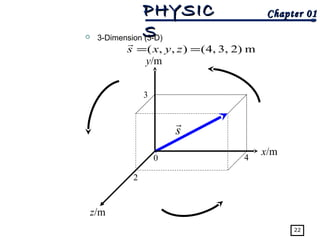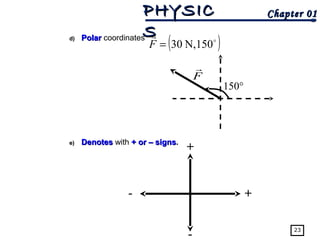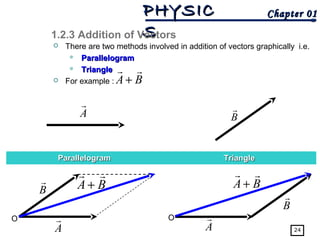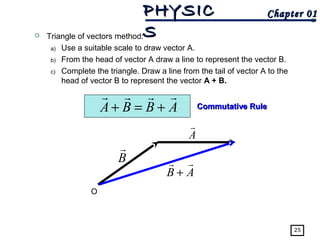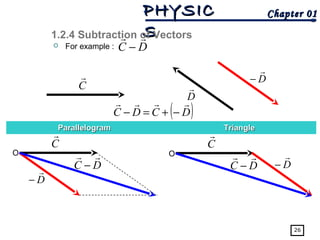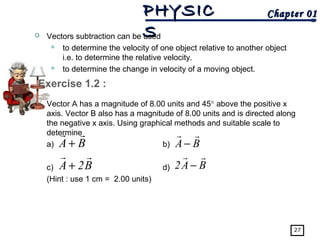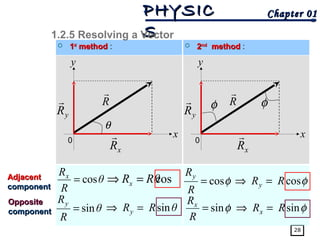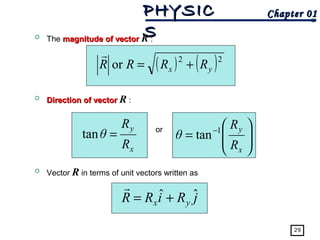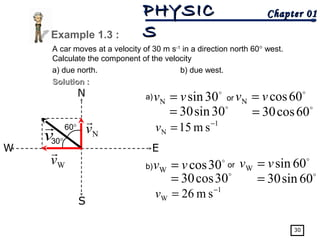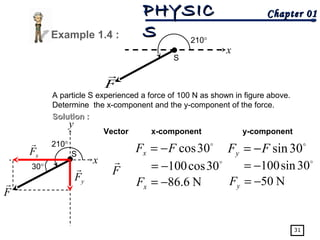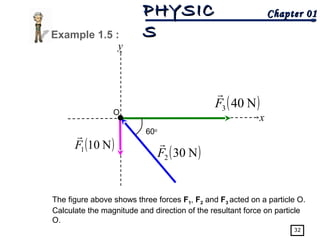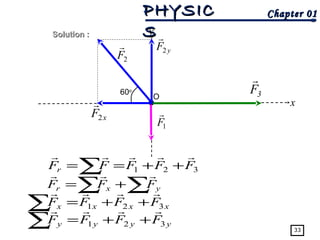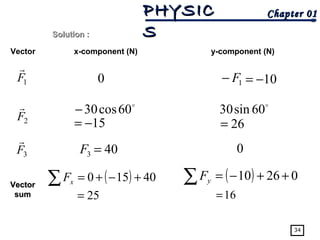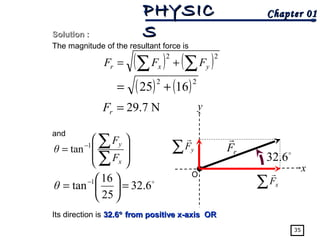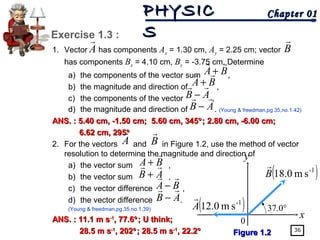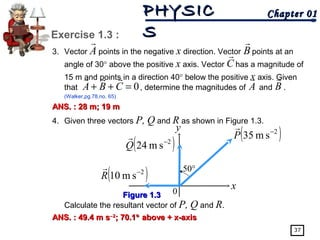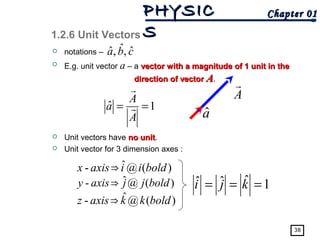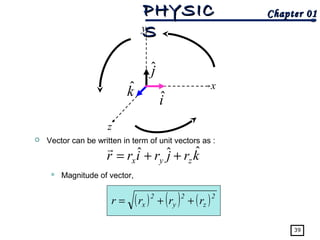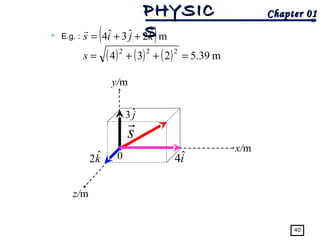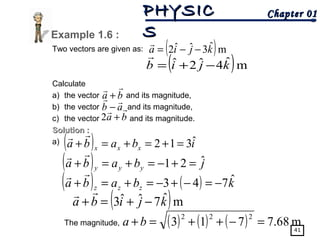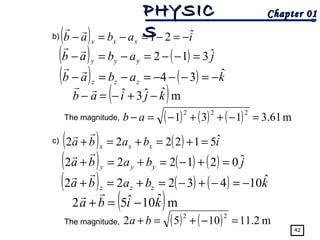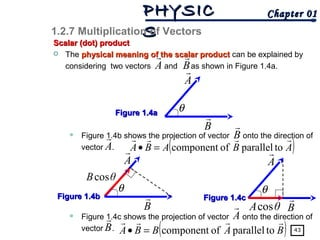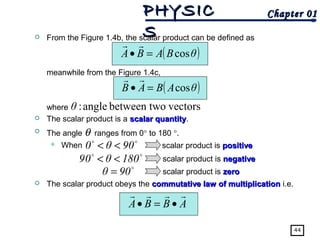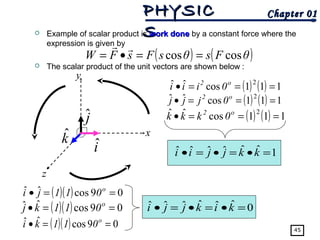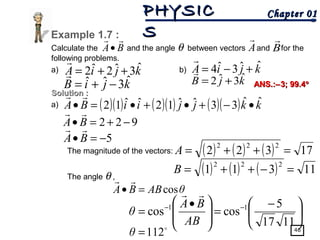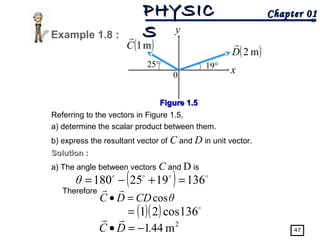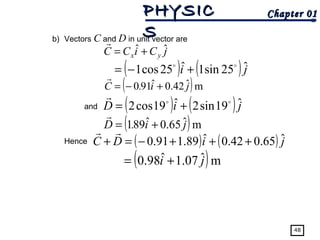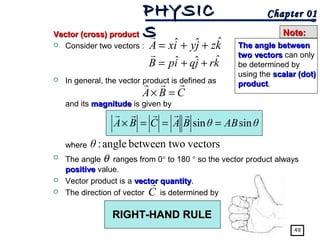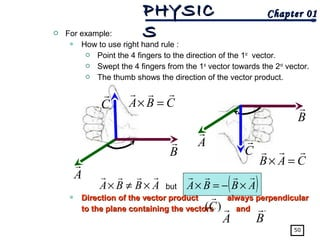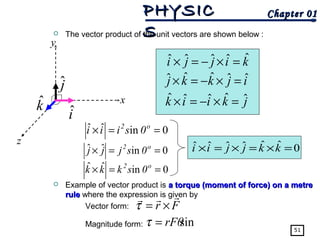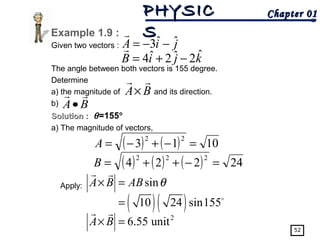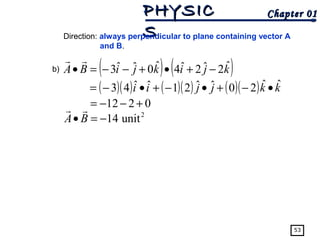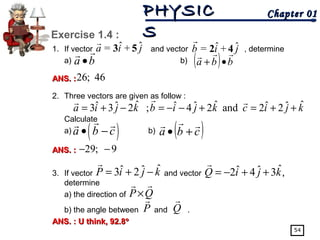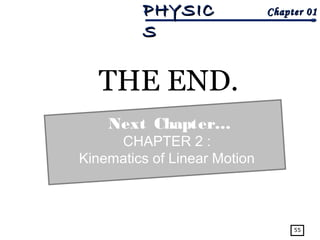1 sur 55

### 1.0 Physical Quantities and Measurement

• 1. Chapter 01Chapter 01PHYSICPHYSIC SS 1 CHAPTER 1:CHAPTER 1: Physical quantities andPhysical quantities and measurementsmeasurements (3 Hours)(3 Hours)
• 2. Chapter 01Chapter 01PHYSICPHYSIC SS 2 Overview: Physical quantitiesPhysical quantities and measurementsand measurements Basic andBasic and derivedderived quantitiesquantities Scalar andScalar and vectorvector quantitiesquantities UnitUnit conversionconversion VectorsVectors multiplicationmultiplication Addition,Addition, subtractionsubtraction and vectorand vector resolutionresolution Scalar andScalar and vectorvector productsproducts
• 3. Chapter 01Chapter 01PHYSICPHYSIC SS 3 At the end of this chapter, students should beAt the end of this chapter, students should be able to:able to:  StateState basic quantities and their respective SIbasic quantities and their respective SI units: length (m), time (s), mass (kg), electricalunits: length (m), time (s), mass (kg), electrical current (A), temperature (K), amount ofcurrent (A), temperature (K), amount of substance (mol) and luminosity (cd).substance (mol) and luminosity (cd).  StateState derived quantities and their respectivederived quantities and their respective units and symbols: velocity (m sunits and symbols: velocity (m s-1-1 ), acceleration), acceleration (m s(m s-2-2 ), work (J), force (N), pressure (Pa), energy), work (J), force (N), pressure (Pa), energy (J), power (W) and frequency (Hz).(J), power (W) and frequency (Hz).  ConvertConvert units to common SI prefixes.units to common SI prefixes. Learning Outcome: 1.1 Physical Quantities and units (1 hour)
• 4. Chapter 01Chapter 01PHYSICPHYSIC SS 4  Physical quantityPhysical quantity is defined as a quantity which can be measured.quantity which can be measured.  It can be categorised into 2 types  Basic (base) quantityBasic (base) quantity  Derived quantityDerived quantity  Basic quantityBasic quantity is defined as a quantity which cannot be derivedquantity which cannot be derived from any physical quantities.from any physical quantities.  Table 1.1 shows all the basic (base) quantities. 1.1 Physical Quantities and Units Quantity Symbol SI Unit Symbol Length l metre m Mass m kilogram kg Time t second s Temperature T/θ kelvin K Electric current I ampere A Amount of substance N mole mol Luminous Intensity candela cdTable 1.1Table 1.1
• 5. Chapter 01Chapter 01PHYSICPHYSIC SS 5  Derived quantityDerived quantity is defined as a quantity which can bequantity which can be derived from basic quantity.derived from basic quantity.  Table 1.2 shows some examples of derived quantity. Derived quantity Symbol Formulae SI Unit Velocity v s/t m s-1 Volume V l × w × t m3 Acceleration a v/t m s-2 Density ρ m/V kg m-3 Momentum p m × v kg m s-1 Force F m × a kg m s-2 @ N Work W F × s kg m2 s-2 @ J Power P W/t kg m2 s-3 @ W Frequency f 1/T s-1 @ Hz Pressure P F/A kg m-1 s-2 @ Pa Table 1.2Table 1.2
• 6. Chapter 01Chapter 01PHYSICPHYSIC SS 6  UnitUnit is defined as a standard size of measurement ofa standard size of measurement of physical quantities.physical quantities.  Examples :  1 second1 second is defined as the time required forthe time required for 9,192,631,770 vibrations of radiation emitted by a9,192,631,770 vibrations of radiation emitted by a caesium-133 atom.caesium-133 atom.  1 kilogram1 kilogram is defined as the mass of a platinum-iridiumthe mass of a platinum-iridium cylinder kept at International Bureau of Weights andcylinder kept at International Bureau of Weights and Measures ParisMeasures Paris.  1 meter1 meter is defined as the length of the path travelled bythe length of the path travelled by light in vacuum during a time interval oflight in vacuum during a time interval of s 458,792,299 1 second kilogram meter
• 7. Chapter 01Chapter 01PHYSICPHYSIC SS The unit of basic quantity is called base unit  addition unit for base unit:  unit of plane angle - radian (rd)  The common system of units used today are S.I unit (S.I unit (SystemSystem International/metric systemInternational/metric system)).  The unit of derived quantity – called derived unit 7 o o o 57.296 180 rad1 180rad == = π π
• 8. Chapter 01Chapter 01PHYSICPHYSIC SS 8  It is used for presenting larger and smaller values.for presenting larger and smaller values.  Table 1.3 shows all the unit prefixes.  Examples:  5740000 m = 5740 km = 5.74 Mm  0.00000233 s = 2.33 × 10−6 s = 2.33 µs Prefix Multiple Symbol tera × 1012 T giga × 109 G mega × 106 M kilo × 103 k deci × 10−1 d centi × 10−2 c milli × 10−3 m micro × 10−6 µ nano × 10−9 n pico × 10−12 p 1.1.1 Unit Prefixes Table 1.3Table 1.3
• 9. Chapter 01Chapter 01PHYSICPHYSIC SS Note:Note: Line of prefix: m, s, N, A, g and etc… T G M k d c m µ n p 1012 109 106 103 100 100 10−1 10−2 10−3 10−6 10−9 10−12 UnitSymbol : Value : How to use?How to use? 1 Ts = ? ps 1 Ts =1012− minus the index - divisionminus the index - division (−12) ps = 1024 ps 9
• 10. Chapter 01Chapter 01PHYSICPHYSIC SS 10 Solve the following problems. a. 45 mm2 = ? m2 b. 37 km h−1 = ? m s−1 c. 30 g cm−3 = ? kg m−3 d. 29 µm = ? m e. 23 m h−1 = ? m s−1 Solution :Solution : a. b. 11stst method :method : 3 1 37 10 m 37 km h 1 h −  × =  ÷   Example 1.1 : 3 37 10 m 3600 s  × =  ÷  1 1 37 km h 10.3 m s− − = 2 2 45 mm 45 1 mm= × ( ) 2 3 45 10 m− = × 2 6 2 5 2 45 mm 45 10 m or 4.5 10 m− − = × × 1.1.2 Conversion of Unit
• 11. Chapter 01Chapter 01PHYSICPHYSIC SS 37 km 1000 m 1 h 1 h 1 km 3600 s     =  ÷ ÷ ÷     11 22ndnd method :method : c. 1 37 km 37 km h 1 h −   =  ÷   1 1 37 km h 10.3 m s− − = ( ) 3 3 3 33 2 3 30 g 10 kg 1 cm 30 g cm 1 cm 1 g 10 m − − −       ÷=  ÷ ÷  ÷     3 4 3 30 g cm 3.0 10 kg m− − = ×
• 12. Chapter 01Chapter 01PHYSICPHYSIC SS 12 d. e. 29 mµ 5 29 m 2.9 10 mµ − = × 1 23 m 1 h 23 m h 1 h 3600 s −    =  ÷ ÷    1 3 1 23 m h 6.39 10 m s− − − = × 6 29 10 m− = × Note:Note:  Unit conversion is important, but it’s also important to recogniserecognise when it’s needed.  Always use the SI unitAlways use the SI unit.
• 13. Chapter 01Chapter 01PHYSICPHYSIC SS 13 Calculate the volume in SI unit of a wire of length 125 cm and diameter 0.65 mm. Solution :Solution : Given l = 1.25 m ; d =0.65×10−3 m The radius of the wire is The volume of the cylindrical wire is given by 3 0.325 10 mr − = × Example 1.2 : ( ) ( ) 2 3 0.325 10 1.25π − = × 7 3 4.15 10 mV − = × 2 V r lπ= 3 0.65 10 2 2 d r − × = =
• 14. Chapter 01Chapter 01PHYSICPHYSIC SS 14 Given 1 (angstrom) Å = 10-10 m 1. Solve all the following problems. 2. Convert the following problems into SI unit. a. 0.249 mm3 b. 5.87 cm2 c. 12 g km h-1 d. 9.78 g cm-3 e. 10 km h-1 f. 8.5 g cm h-2 Exercise 1.1 : a. 35 cm =_________m f. 24 mm2 =___________m2 b. 20 km =_________m g. 0.03 m2 =____________ mm2 c. 11 Mm =_________m h. 19 km2 =_____________ m2 d. 23 µF =_________F i. 56 g cm-3 =_________ kg m-3 e. 0.24 Å =_________m j. 560 km h-1 =_________ m s-1
• 15. Chapter 01Chapter 01PHYSICPHYSIC SS 15 At the end of this chapter, students should be able to:At the end of this chapter, students should be able to:  DefineDefine scalar and vector quantities.scalar and vector quantities.  PerformPerform vector addition and subtraction graphically.vector addition and subtraction graphically.  Resolve vectorResolve vector into two perpendicular components(x andinto two perpendicular components(x and y axes)y axes)  Ilustrate unit vectors in cartesion coordinate.Ilustrate unit vectors in cartesion coordinate.  State the physical meaning of dot(scalar) product:State the physical meaning of dot(scalar) product:   State the physical meaning of cross(vector) product:State the physical meaning of cross(vector) product:  Direction of cross product is determined by corkscrewDirection of cross product is determined by corkscrew method or right hand rule.method or right hand rule. Learning Outcome: 1.2 Scalars and Vectors (2 hours) ( , , )i j k%% % ( cos ) ( cos )A B A B B Aθ θ• = = r r ( sin ) ( sin )A B A B B Aθ θ× = = r r
• 16. Chapter 01Chapter 01PHYSICPHYSIC SS 16  State the physical meaning of dot(scalar)State the physical meaning of dot(scalar) product:product:  State the physical meaning of cross(vector)State the physical meaning of cross(vector) product:product: Direction of cross product is determined byDirection of cross product is determined by corkscrew method or right hand rule.corkscrew method or right hand rule. Learning Outcome: 1.2 Scalars and Vectors (2 hours) ( ) ( )θABθBABA coscos ==• rr ( ) ( )θABθBABA sinsin ==× rr
• 17. Chapter 01Chapter 01PHYSICPHYSIC SS 17  ScalarScalar quantity is defined as a quantity with magnitudequantity with magnitude only.  e.g. mass, time, temperature, pressure, electric current, work, energy, power and etc.  Mathematics operational : ordinary algebra  VectorVector quantity is defined as a quantity with both magnitudequantity with both magnitude & direction.& direction.  e.g. displacement, velocity, acceleration, force, momentum, electric field, magnetic field and etc.  Mathematics operational : vector algebra 1.2 Scalars and Vectors
• 18. Chapter 01Chapter 01PHYSICPHYSIC SS 18  Table 1.4 shows written form (notation) of vectors.  Notation of magnitude of vectors. 1.2.1 Vectors Vector A LengthLength of an arrow– magnitudemagnitude of vector A displacement velocity acceleration s r v r a r s av vv = r aa = r s (bold) v (bold) a (bold) DirectionDirection of arrow – directiondirection of vector A Table 1.4Table 1.4
• 19. Chapter 01Chapter 01PHYSICPHYSIC SS 19  Two vectorsTwo vectors equal if both magnitude and directionmagnitude and direction are the same.same. (shown in figure 1.1)  If vector A is multiplied by a scalar quantity k  Then, vector A is  if kk = +ve= +ve, the vector is in the same directionsame direction as vector A.  if kk == --veve, the vector is in the opposite directionopposite direction of vector A. P r Q r QP rr = Figure 1.1Figure 1.1 Ak r Ak r A r A r −
• 20. Chapter 01Chapter 01PHYSICPHYSIC SS 20  Can be represented by using: a)a) Direction of compassDirection of compass, i.e east, west, north, south, north-east, north-west, south-east and south-west b)b) Angle with a reference lineAngle with a reference line e.g. A boy throws a stone at a velocity of 20 m s-1 , 50° above horizontal. 1.2.2 Direction of Vectors 50° v r x y 0
• 21. Chapter 01Chapter 01PHYSICPHYSIC SS 21 c)c) CartesianCartesian coordinates  2-Dimension (2-D) m)5m,1(),( == yxs r s r y/m x/m 5 10
• 22. Chapter 01Chapter 01PHYSICPHYSIC SS 22  3-Dimension (3-D) s r 2 3 4 m2)3,4,(),,( == zyxs r y/m x/m z/m 0
• 23. Chapter 01Chapter 01PHYSICPHYSIC SS 23 d)d) PolarPolar coordinates e)e) DenotesDenotes with + or – signs+ or – signs. ( ) r N,15030=F F r 150° + +- -
• 24. Chapter 01Chapter 01PHYSICPHYSIC SS 24  There are two methods involved in addition of vectors graphically i.e.  ParallelogramParallelogram  TriangleTriangle  For example : 1.2.3 Addition of Vectors ParallelogramParallelogram TriangleTriangle B r A r B r A r BA rr + O BA rr + B r A r BA rr + O
• 25. Chapter 01Chapter 01PHYSICPHYSIC SS 25  Triangle of vectors method: a) Use a suitable scale to draw vector A. b) From the head of vector A draw a line to represent the vector B. c) Complete the triangle. Draw a line from the tail of vector A to the head of vector B to represent the vector A + B. ABBA rrrr +=+ Commutative RuleCommutative Rule B r A r AB rr + O
• 26. Chapter 01Chapter 01PHYSICPHYSIC SS 26  For example : 1.2.4 Subtraction of Vectors ParallelogramParallelogram TriangleTriangle D rC r O DC rr − O D r − ( )DCDC rrrr −+=− C r D r − DC rr − C r D r −DC rr −
• 27. Chapter 01Chapter 01PHYSICPHYSIC SS 27  Vectors subtraction can be used  to determine the velocity of one object relative to another object i.e. to determine the relative velocity.  to determine the change in velocity of a moving object. 1. Vector A has a magnitude of 8.00 units and 45° above the positive x axis. Vector B also has a magnitude of 8.00 units and is directed along the negative x axis. Using graphical methods and suitable scale to determine a) b) c) d) (Hint : use 1 cm = 2.00 units) Exercise 1.2 : BA rr + BA rr − B2A rr + BA2 rr −
• 28. Chapter 01Chapter 01PHYSICPHYSIC SS 28  11stst methodmethod : 1.2.5 Resolving a Vector R r yR r xR r θ 0 x y θ R Rx cos= cosxR Rθ⇒ = θ R Ry sin= θRRy sin=⇒  22ndnd methodmethod : R r yR r xR r φ 0 x y φsin= R Rx φsinRRx =⇒ φcos= R Ry φcosRRy =⇒ φ AdjacentAdjacent componentcomponent OppositeOpposite componentcomponent
• 29. Chapter 01Chapter 01PHYSICPHYSIC SS 29  The magnitude of vectormagnitude of vector R :  Direction of vectorDirection of vector R :  Vector R in terms of unit vectors written as ( ) ( )22 or yx RRRR +=  x y R R θ =tan or       = − x y R R θ 1 tan jRiRR yx ˆˆ += 
• 30. Chapter 01Chapter 01PHYSICPHYSIC SS 30 A car moves at a velocity of 30 m s-1 in a direction north 60° west. Calculate the component of the velocity a) due north. b) due west. Solution :Solution : Example 1.3 : N EW S Nv  v  a) b)  60cosN vv = 1 N sm51 − =v  60cos30= or  30sinN vv =  30sin30=  60sinW vv = 1 W sm26 − =v  60sin30= or  30cosW vv =  30cos30= 60° 30° Wv 
• 31. Chapter 01Chapter 01PHYSICPHYSIC SS 31 A particle S experienced a force of 100 N as shown in figure above. Determine the x-component and the y-component of the force. Solution :Solution : Example 1.4 : Vector x-component y-component  30cosFFx −= N6.68−=xF  30cos100−=F   30sinFFy −= N05−=yF  30sin100−= 210° F  S x S 210° F  x y 30° xF  yF 
• 32. Chapter 01Chapter 01PHYSICPHYSIC SS 32 The figure above shows three forces F1, F2 and F3 acted on a particle O. Calculate the magnitude and direction of the resultant force on particle O. Example 1.5 : y O 60o x ( )N101F  ( )N302F  ( )N403F 
• 33. Chapter 01Chapter 01PHYSICPHYSIC SS x y O 3F  33 Solution :Solution : ∑ ++== 321 FFFFFr  ∑ ∑+= yxr FFF  xxxx FFFF 321  ++=∑ yyyy FFFF 321  ++=∑ 2F  1F  60o xF2  yF2 
• 34. Chapter 01Chapter 01PHYSICPHYSIC SS 34 Solution :Solution : Vector x-component (N) y-component (N) 1F  3F  2F  0 1F− 01−=  60cos30− 15−=  60sin30 62= 3F 40= 0 VectorVector sumsum ( ) 40510 +−+=∑ xF 25= ( ) 02601 ++−=∑ yF 16=
• 35. Chapter 01Chapter 01PHYSICPHYSIC SSSolution :Solution : The magnitude of the resultant force is and Its direction is 32.632.6°° from positive x-axis ORfrom positive x-axis OR 35 y x O ( ) ( )22 ∑∑ += yxr FFF N.729=rF ( ) ( )22 1625 +=         = ∑ ∑− x y F F θ 1 tan  6.32 25 16 tan 1 =      = − θ ∑ yF  rF   32.6 ∑ xF 
• 36. Chapter 01Chapter 01PHYSICPHYSIC SS 36 1. Vector has components Ax = 1.30 cm, Ay = 2.25 cm; vector has components Bx = 4.10 cm, By = -3.75 cm. Determine a) the components of the vector sum , b) the magnitude and direction of , c) the components of the vector , d) the magnitude and direction of . (Young & freedman,pg.35,no.1.42) ANS. : 5.40 cm, -1.50 cm; 5.60 cm, 345ANS. : 5.40 cm, -1.50 cm; 5.60 cm, 345°°; 2.80 cm, -6.00 cm;; 2.80 cm, -6.00 cm; 6.62 cm, 2956.62 cm, 295°° 2. For the vectors and in Figure 1.2, use the method of vector resolution to determine the magnitude and direction of a) the vector sum , b) the vector sum , c) the vector difference , d) the vector difference . (Young & freedman,pg.35,no.1.39) ANS. : 11.1 m sANS. : 11.1 m s-1-1 , 77.6, 77.6°°; U think;; U think; 28.5 m s28.5 m s-1-1 , 202, 202°°; 28.5 m s; 28.5 m s-1-1 , 22.2, 22.2°° Exercise 1.3 : BA  + A  BA  + AB  − AB  − B  A  B  BA  + AB  + BA  − AB  − Figure 1.2Figure 1.2 y x 0 37.0° ( )-1 sm18.0B  ( )-1 sm12.0A 
• 37. Chapter 01Chapter 01PHYSICPHYSIC SS 37 3. Vector points in the negative x direction. Vector points at an angle of 30° above the positive x axis. Vector has a magnitude of 15 m and points in a direction 40° below the positive x axis. Given that , determine the magnitudes of and . (Walker,pg.78,no. 65) ANS. : 28 m; 19 mANS. : 28 m; 19 m 4. Given three vectors P, Q and R as shown in Figure 1.3. Calculate the resultant vector of P, Q and R. ANS. : 49.4 m sANS. : 49.4 m s−−22 ; 70.1; 70.1°° above + x-axisabove + x-axis Exercise 1.3 : C A  B  0=++ CBA  A  B  Figure 1.3Figure 1.3 y x0 50° ( )2 sm10 − R  ( )2 sm35 − P  ( )2 sm24 − Q 
• 38. Chapter 01Chapter 01PHYSICPHYSIC SS 38  notations –  E.g. unit vector a – a vector with a magnitude of 1 unit in thevector with a magnitude of 1 unit in the direction of vectordirection of vector AA.  Unit vectors have no unitno unit.  Unit vector for 3 dimension axes : 1.2.6 Unit Vectors A  aˆ cba ˆ,ˆ,ˆ 1ˆ == A A a   )(@ˆ⇒- boldjjaxisy 1ˆˆˆ === kji )(@ˆ⇒- boldiiaxisx )(@ˆ⇒- boldkkaxisz
• 39. Chapter 01Chapter 01PHYSICPHYSIC SS 39  Vector can be written in term of unit vectors as :  Magnitude of vector, x z y kˆ jˆ iˆ krjrirr zyx ˆˆˆ ++=  ( ) ( ) ( )2 z 2 y 2 x rrrr ++=
• 40. Chapter 01Chapter 01PHYSICPHYSIC SS 40  E.g. : ( )mˆ2ˆ3ˆ4 kjis ++=  ( ) ( ) ( ) m5.39234 222 =++=s jˆ3 x/m y/m z/m 0 s  iˆ4kˆ2
• 41. Chapter 01Chapter 01PHYSICPHYSIC SS 41 Two vectors are given as: Calculate a) the vector and its magnitude, b) the vector and its magnitude, c) the vector and its magnitude. Solution :Solution : a) The magnitude, Example 1.6 : ab  − ( )mˆ3ˆˆ2 kjia −−=  ba  + ( )mˆ4ˆ2ˆ kjib −+=  ( ) ibaba xxx ˆ312 =+=+=+  ( ) jbaba yyy ˆ21 =+−=+=+  ( )mˆ7ˆˆ3 kjiba −+=+  ( ) ( ) kbaba zzz ˆ743 −=−+−=+=+  ( ) ( ) ( ) m68.7713 222 =−++=+ ba ba  +2
• 42. Chapter 01Chapter 01PHYSICPHYSIC SS 42 b) The magnitude, c) The magnitude, ( ) iabab xxx ˆ21 −=−=−=−  ( ) ( ) jabab yyy ˆ312 =−−=−=−  ( )mˆˆ3ˆ kjiab −+−=−  ( ) ( ) kabab zzz ˆ34 −=−−−=−=−  ( ) ( ) ( ) m.613131 222 =−++−=− ab ( ) ( ) ibaba xxx ˆ512222 =+=+=+  ( ) ( ) ( ) jbaba yyy ˆ021222 =+−=+=+  ( )mˆ10ˆ52 kiba −=+  ( ) ( ) ( ) kbaba zzz ˆ1043222 −=−+−=+=+  ( ) ( ) m11.21052 22 =−+=+ ba
• 43. Chapter 01Chapter 01PHYSICPHYSIC SS 43 Scalar (dot) productScalar (dot) product  The physical meaning of the scalar productphysical meaning of the scalar product can be explained by considering two vectors and as shown in Figure 1.4a.  Figure 1.4b shows the projection of vector onto the direction of vector .  Figure 1.4c shows the projection of vector onto the direction of vector . 1.2.7 Multiplication of Vectors A  B  θ A  B  A  B  Figure 1.4aFigure 1.4a θ A  B  A  B  θBcos Figure 1.4bFigure 1.4b θ A  B  θAcos Figure 1.4cFigure 1.4c ( )ABABA  toparallelofcomponent=• ( )BABBA  toparallelofcomponent=•
• 44. Chapter 01Chapter 01PHYSICPHYSIC SS 44  From the Figure 1.4b, the scalar product can be defined as meanwhile from the Figure 1.4c, where  The scalar product is a scalar quantityscalar quantity.  The angle θ ranges from 0° to 180 °.  When  The scalar product obeys the commutative law of multiplicationcommutative law of multiplication i.e. ( )θBABA cos=•  vectorsobetween twangle:θ ( )θABAB cos=•   90θ0 << scalar product is positivepositive  180θ09 << scalar product is negativenegative  90θ = scalar product is zerozero ABBA  •=•
• 45. Chapter 01Chapter 01PHYSICPHYSIC SS 45  Example of scalar product is work donework done by a constant force where the expression is given by  The scalar product of the unit vectors are shown below : ( ) ( )θFsθsFsFW coscos ==•=  x z y kˆ jˆ iˆ ( ) ( ) 111cosˆˆ 2 ===• o2 0iii 1ˆˆˆˆˆˆ =•=•=• kkjjii ( ) ( ) 111cosˆˆ 2 ===• o2 0jjj ( ) ( ) 111cosˆˆ 2 ===• o2 0kkk ( )( ) 09cosˆˆ ==• o 011ji 0ˆˆˆˆˆˆ =•=•=• kikjji ( )( ) 09cosˆˆ ==• o 011ki ( )( ) 09cosˆˆ ==• o 011kj
• 46. Chapter 01Chapter 01PHYSICPHYSIC SS 46 Calculate the and the angle θ between vectors and for the following problems. a) b) Solution :Solution : a) The magnitude of the vectors: The angle θ , Example 1.7 : A  BA  • B  ( )( ) ( )( ) ( )( ) kkjjiiBA ˆˆ33ˆˆ12ˆˆ12 •−+•+•=•  ( ) ( ) ( ) 17322 222 =++=A kjiA ˆ3ˆ2ˆ2 ++=  kjiA ˆˆ3ˆ4 +−=  kjiB ˆ3ˆˆ −+=  kjB ˆ3ˆ2 +=  922 −+=• BA  5−=• BA  ( ) ( ) ( ) 11311 222 =−++=B θABBA cos=•        − =        • = −− 1117 5 coscos 11 AB BA θ   112=θ ANS.:ANS.:−−3; 99.43; 99.4°°
• 47. Chapter 01Chapter 01PHYSICPHYSIC SS 47 Referring to the vectors in Figure 1.5, a) determine the scalar product between them. b) express the resultant vector of C and D in unit vector. Solution :Solution : a) The angle between vectors C and D is Therefore Example 1.8 : 2 m441.DC −=•  ( )  1361925180 =+−=θ Figure 1.5Figure 1.5 y x0 ( )m1C  ( )m2D  19°25° θCDDC cos=•  ( )( )  136cos21=
• 48. Chapter 01Chapter 01PHYSICPHYSIC SS 48 b) Vectors C and D in unit vector are and Hence jCiCC yx ˆˆ +=  ( ) ( )ji ˆ25sin1ˆ25cos1  +−= ( )mˆ42.0ˆ910 ji.C +−=  ( ) ( ) jiDC ˆ65.042.0ˆ89.191.0 +++−=+  ( )mˆ07.1ˆ98.0 ji += ( ) ( )jiD ˆ19sin2ˆ19cos2   += ( )mˆ65.0ˆ891 ji.D += 
• 49. Chapter 01Chapter 01PHYSICPHYSIC SS 49 Vector (cross) productVector (cross) product  Consider two vectors :  In general, the vector product is defined as and its magnitudemagnitude is given by where  The angle θ ranges from 0° to 180 ° so the vector product always positivepositive value.  Vector product is a vector quantityvector quantity.  The direction of vector is determined by krjqipB ˆˆˆ ++=  kzjyixA ˆˆˆ ++=  CBA  =× θABθBACBA sinsin ===×  vectorsobetween twangle:θ RIGHT-HAND RULERIGHT-HAND RULE C  Note:Note: The angle betweenThe angle between two vectorstwo vectors can only be determined by using the scalar (dot)scalar (dot) productproduct.
• 50. Chapter 01Chapter 01PHYSICPHYSIC SS 50  For example:  How to use right hand rule :  Point the 4 fingers to the direction of the 1st vector.  Swept the 4 fingers from the 1st vector towards the 2nd vector.  The thumb shows the direction of the vector product.  Direction of the vector product always perpendicularDirection of the vector product always perpendicular to the plane containing the vectors andto the plane containing the vectors and . A  C  B  A  B  C  CBA  =× CAB  =× ABBA  ×≠× but ( )ABBA  ×−=× B )(C  A 
• 51. Chapter 01Chapter 01PHYSICPHYSIC SS 51  The vector product of the unit vectors are shown below :  Example of vector product is a torque (moment of force) on a metrea torque (moment of force) on a metre rulerule where the expression is given by Vector form: Magnitude form: x z y kˆ jˆ iˆ ijkkj ˆˆˆˆˆ =×−=× kijji ˆˆˆˆˆ =×−=× jkiik ˆˆˆˆˆ =×−=× 0ˆˆˆˆˆˆ =×=×=× kkjjii 0inˆˆ ==× o2 0siii 0inˆˆ ==× o2 0sjjj 0inˆˆ ==× o2 0skkk r Fτ = ×   sinrFθτ =
• 52. Chapter 01Chapter 01PHYSICPHYSIC SS 52 Given two vectors : The angle between both vectors is 155 degree. Determine a) the magnitude of and its direction. b) Solution :Solution : θ=155° a) The magnitude of vectors, Apply: Example 1.9 : BA  × BA  • jiA ˆˆ3 −−=  kjiB ˆ2ˆ2ˆ4 −+=  sinA B AB θ× =   ( ) ( ) 1013 22 =−+−=A ( ) ( ) ( ) 24224 222 =−++=B ( )( )10 24 sin155=  2 6.55 unitA B× =  
• 53. Chapter 01Chapter 01PHYSICPHYSIC SS 53 Direction: always perpendicular to plane containing vector A and B. b) ( ) ( )kjikjiBA ˆ2ˆ2ˆ4ˆ0ˆˆ3 −+•+−−=•  2 14 unitA B• = −   ( )( ) ( )( ) ( )( ) kkjjii ˆˆ20ˆˆ21ˆˆ43 •−+•−+•−= 0212 +−−=
• 54. Chapter 01Chapter 01PHYSICPHYSIC SS 54 1. If vector and vector , determine a) b) ANS. :ANS. : 2. Three vectors are given as follow : Calculate a) b) ANS. :ANS. : 3. If vector and vector , determine a) the direction of b) the angle between and . ANS. : U think, 92.8ANS. : U think, 92.8°° Exercise 1.4 : 26; 46 jia ˆ+ˆ= 53  jib ˆ+ˆ= 42  ba  • ( ) bba  •+ kjickjibkjia ˆˆ2ˆ2andˆ2ˆ4ˆ;ˆ2ˆ3ˆ3 ++=+−−=−+=  ( )a b c• −   ( )cba  +• 29; 9− − kjiP ˆˆ2ˆ3 −+=  kjiQ ˆ3ˆ4ˆ2 ++−=  QP  × P  Q 
• 55. Chapter 01Chapter 01PHYSICPHYSIC SS 55 THE END. Next Chapter… CHAPTER 2 : Kinematics of Linear Motion